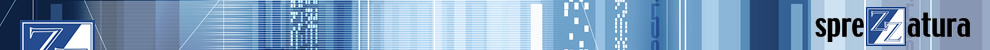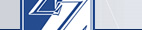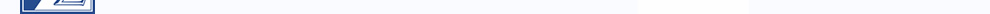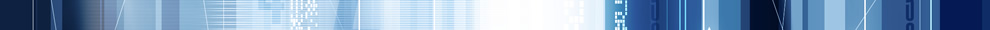In order for this site to work correctly, and for us to improve the site, we need to store a small file (called a cookie) on your computer.# RevMedia

1. Volume 1
2. Volume 2
3. Volume 3
4. Volume 4

### Uncommon Knowledge - WC_Delete_Rec%

A flag variable used to tell the system that the current record ought to be deleted (when the variable is set to TRUE).

To force the system to recognise that WC_Delete_Rec% has been set, the following action must be taken (remember that different values of WC_Reset% have different effects on WC_Is% and WC_Is_Orig% ):-

```Default        Setting WC_Reset% to 4 will run the Pre Prompt logic followed
by the Perpetual logic and, if WC_Reset% is not changed, will
carry on to delete the record (and thus call all Delete
related logic (pre, replace and post)). It will then return
to the key prompt. Setting WC_Reset% to 5 will repeat the
above but omit the Perpetual logic.

In the special case where WC_Delete_rec% is set on the first
time through the pre prompt on the first prompt on the
screen, the above logic will be followed but immediately
after the post delete process, the system will continue on
through the read processes (pre. rep and post) using the @ID
just deleted, thus it will report that it is adding a new
record. Note that this will not occur where the window is
invoked with the current @ID on the command line (EG WINDOW
TEST 1), only if the current @ID is actually entered into the
window.

Pre Prompt     Setting WC_Reset% to 4 will run the Perpetual logic and, if
WC_Reset% is not changed, will delete the record (and thus
call all Delete related logic (pre, replace and post)). It
will repeat the above but omit the Perpetual logic.

In the special case where WC_Delete_rec% is set on the first
time through the pre prompt on the first prompt on the
screen, the logic described above for 'special case' default
will be followed, though omitting calling the pre prompt
logic.

Options        Setting WC_Reset% to 2 or 3 will run the Post logic followed
by the Perpetual logic and, if WC_Reset% is not changed, will
delete the record (and thus call all Delete related logic
prompt. Setting WC_Reset% to 4 will run the Perpetual logic
and, if WC_Reset% is not changed, will delete the record (and
thus call all Delete related logic (pre, replace and post)).
It will then return to the key prompt. Setting WC_Reset% to 5
will delete the record (and thus call all Delete related
key prompt.

Edit           WC_Reset% values of 0 and 1 will be ignored. WC_Reset% values
of 2 and 3 will run Post logic then Perpetual logic and, if
WC_Reset% is not changed, will delete the record (and thus
call all Delete related logic (pre, replace and post)). It
will then return to the key prompt. A WC_Reset% value of 4
will run the Perpetual logic and, if WC_Reset% is not changed
, will delete the record (and thus call all Delete related
key prompt. A WC_Reset% value of 5 will delete the record
(and thus call all Delete related logic (pre, replace and

Post           No action necessary. Will run Perpetual logic and, if
WC_Reset% is not changed, will delete the record (and thus
call all Delete related logic (pre, replace and post)). It
will behave as above but omit the Perpetual logic.

Invalid        Setting WC_Reset% to a number between 2 and 5 will reprompt
for the information. If the Edit logic does not then change
WC_Reset% the following will occur. Setting WC_Reset% to 2 or
3 will run the Post logic followed by the Perpetual logic
and, if WC_Reset% is not changed, will delete the record
(and thus call all Delete related logic (pre, replace and
WC_Reset% to 4 will run the Perpetual logic and, if WC_Reset%
is not changed, will delete the record (and thus call all
Delete related logic (pre, replace and post)). It will then
behave as for WC_Reset% = 4  but omit the Perpetual logic.

Softkeys       Setting WC_Reset% to 2 or 3 will run the Post logic followed
by the Perpetual logic and, if WC_Reset% is not changed, will
delete the record (and thus call all Delete related logic
prompt. Setting WC_Reset% to 4 will run the Perpetual logic
and,  if WC_Reset% is not changed, will delete the record
(and thus call all Delete related logic (pre, replace and